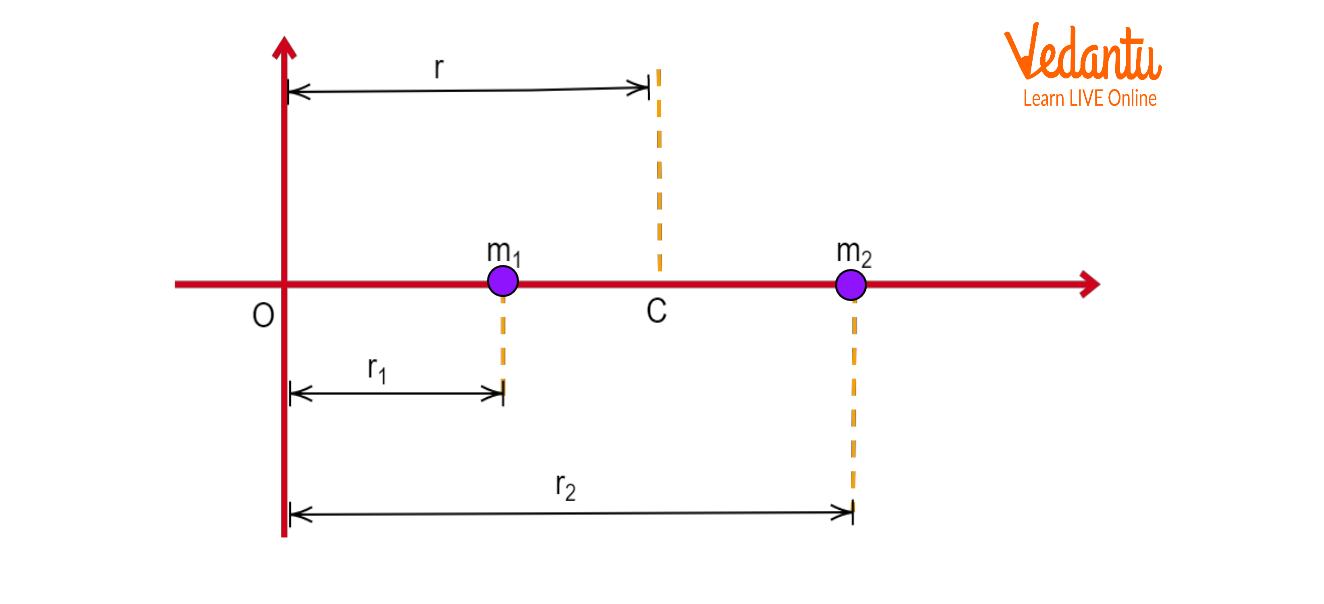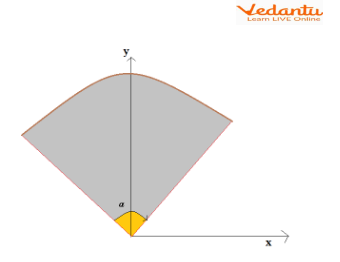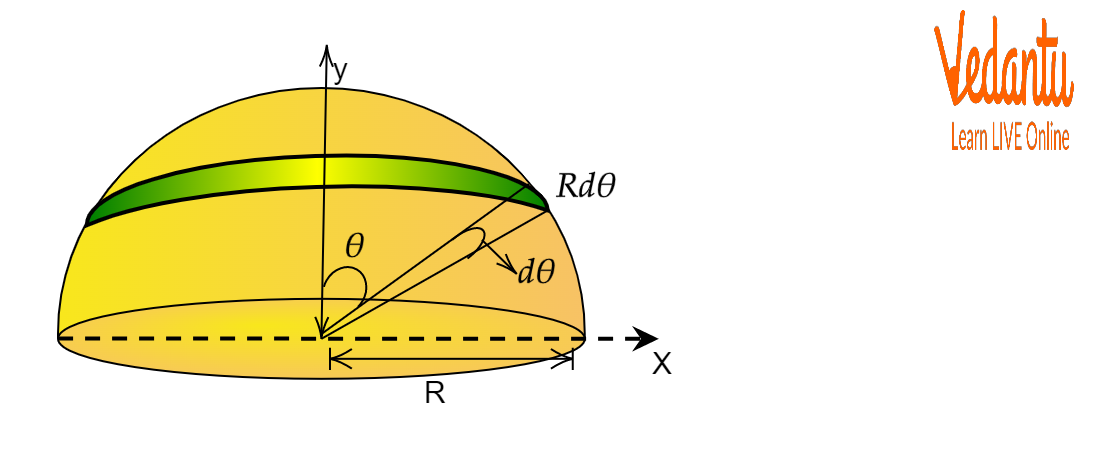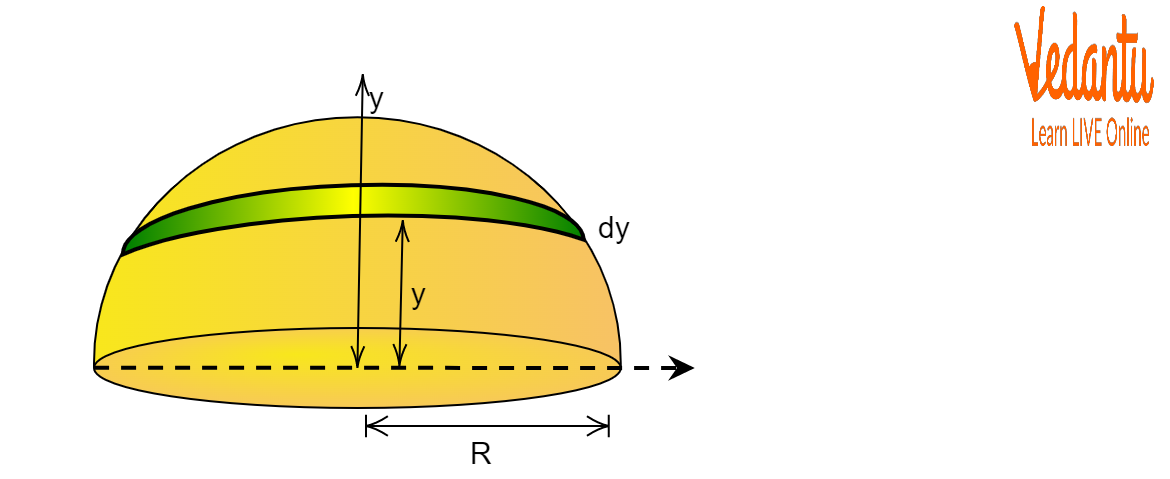Courses
Courses for Kids
Free study material
Free LIVE classes
More

# Centre of Mass of Hollow and Solid Hemisphere - JEE Important TopicLIVE
Join Vedantu’s FREE Mastercalss

## What is the Centre of Mass?

The centre of mass in Physics is explained as the point where the weighted position relative to other points of the mass of a body becomes zero, making it a point of balance. A mass in an object can be distributed arbitrarily. So basically it is a hypothetical position defined in physics itself relative to any arbitrary object or even a system of objects based on the mass distribution. At this point, force can be applied to impart the body with some linear acceleration without any angular acceleration. If a body is pivoted through the centre of mass then only the effects of rotation can be seen.

### Derivation of Centre of Mass

First of all, it has to be kept in mind that now the motion of a single particle is not the only concern. Now a system of particles has to be studied which constitutes a rigid body. In a rigid body, the assembly of particles has a certain finite distance between the particles. So, for these rigid bodies there is a point where the entire mass is supposed to be concentrated.

Let us derive the centre of mass of a simplified two particle system.Derivation of Centre of Mass

From the figure, we see two masses m1 and m2 at distances r1 and r2 respectively from the origin O. Let us assume that the centre of mass of the system lies at point C which is at distance r from origin O.

Therefore, the weighted mean at C is given as

$r=\frac{{{\text{m}}_{\text{1}}}{{\text{r}}_{\text{1}}}\text{+}{{\text{m}}_{\text{2}}}{{\text{r}}_{\text{2}}}}{{{\text{m}}_{\text{1}}}\text{+}{{\text{m}}_{\text{2}}}}$

In vector form, this can be written as

${{\vec{r}}_{cm}}=\dfrac {{m_1}{\vec {r_1}}+{m_2}{\vec {r_2}}}{{m_1}+{m_2}}$

Therefore, this simplified system can be generalised to an n-particle system.

${{\vec{r}}_{cm}}=\dfrac {{m_1}{\vec {r_1}}+{m_2}{\vec {r_2}}+.......+{m_n}{\vec {r_n}}}{{m_1}+{m_2}+.....+{m_n}}$

Or, the compact form is given as

${{\vec{r}}_{cm}}=\frac{1}{M}\sum\limits_{i=1}^{n}{{{m}_{i}}{{{\vec{r}}}_{i}}}$

The above expression is for discrete particle distribution in rigid bodies. Now, for continuous distribution, we can replace the summation sign with integration sign.

${{\vec{r}}_{cm}}=\frac{1}{M}\int{\vec{r}dm}$

In many particle systems, it is convenient to calculate the centre of mass in x, y and z direction in the Cartesian coordinate system.

${{X}_{CM}}=\text{ }\frac{1}{M}\sum\nolimits_{i=1}^{n}{{{m}_{i}}{{x}_{i}}}$

${{Y}_{CM}}=\text{ }\frac{1}{M}\sum\nolimits_{i=1}^{n}{{{m}_{i}}{{y}_{i}}}$

${{Z}_{CM}}=\text{ }\frac{1}{M}\sum\nolimits_{i=1}^{n}{{{m}_{i}}{{z}_{i}}}$

## Centre of Mass of Some Symmetric Figures

The centre of masses of some symmetric figures is given below.

1. Equilateral TriangleAn Equilateral Triangle

The centre of mass is at the centroid that is at a distance of $\frac{\text{H}}{\text{3}}$ from the base.

1. Uniform circular arc or ringUniform Circular arc or Ring

${{x}_{CM}} = 0$, due to the symmetry of the arc along y-axis

${{y}_{CM}}=\frac{R\sin \left( \frac{\alpha }{2} \right)}{\left( \frac{\alpha }{2} \right)}$

1. Semicircular DiscCentre of Mass of Semicircular Disc

Again, for this case;

${{x}_{CM}}=0$, due to the symmetry of the arc along y-axis

${{y}_{CM}}=\frac{4R}{3\pi }$

Now, we will next discuss the COM of hollow and solid hemispheres.

## Centre of Mass of Hollow Hemisphere

Let us derive the centre of mass of a hollow hemisphere. We consider a hollow hemisphere of mass M and radius R. The position where the centre of mass lies is on the y-axis, which is the line passing through the middle point in the base of the hemisphere.Centre of Mass of Hollow Hemisphere

Derivation of com of solid hemisphere

Let’s consider an elemental strip of mass $\text{dM}$  and has a width of $\text{Rd }\!\!\theta\!\!\text{ }$. Therefore, the length of the radius of the hollow hemisphere is $\text{r}\ \text{=}\ \text{sin }\!\!\theta\!\!\text{ }$.

Now we have to find the expression for $\text{dM}$,

$\text{dM}\ \text{=}\ \left( \frac{\text{M}}{\text{2 }\!\!\pi\!\!\text{ }{{\text{R}}^{\text{2}}}} \right)\left( \text{2 }\!\!\pi\!\!\text{ Rsin }\!\!\theta\!\!\text{ }\text{.Rd }\!\!\theta\!\!\text{ } \right)$

$\text{=}\ \text{Msin }\!\!\theta\!\!\text{ d }\!\!\theta\!\!\text{ }$

Now, along y-axis, the y coordinate of COM is given as

${{y}_{CM}}=\frac{1}{M}\int{yDM}$

Substitute the values of $\text{dM}$ and $\text{y}\ \text{=}\ \text{Rcos }\!\!\theta\!\!\text{ }$ in the above equation, we get

${{\text{y}}_{\text{CM}}}\ \text{=}\ \frac{\text{1}}{\text{M}}\int{\left( \text{Rcos }\!\!\theta\!\!\text{ } \right)}\left( \text{Msin }\!\!\theta\!\!\text{ d }\!\!\theta\!\!\text{ } \right)$

${{\text{y}}_{\text{CM}}}\ \text{=}\ \frac{\text{1}}{\text{M}}\int\limits_{\text{0}}^{\frac{\text{ }\!\!\pi\!\!\text{ }}{\text{2}}}{\left( \text{Rcos }\!\!\theta\!\!\text{ } \right)}\left( \text{Msin }\!\!\theta\!\!\text{ d }\!\!\theta\!\!\text{ } \right)$

${{\text{y}}_{\text{CM}}}\ \text{=}\ \frac{\text{R}}{\text{2}}\int\limits_{\text{0}}^{\frac{\text{ }\!\!\pi\!\!\text{ }}{\text{2}}}{\left( \text{sin2 }\!\!\theta\!\!\text{ } \right)}$

$= \frac{R}{2}\left( \frac{1}{2}+\frac{1}{2} \right)$

$= \frac{R}{2}$

Therefore, the centre of mass of a hollow hemisphere is at $\frac{R}{2}$ on the y-axis.

## COM of Solid Hemisphere

The same treatment as above can be applied here to find the COM. Here, we take a solid hemisphere of radius R and mass M with its centre of mass lying on the vertical axis passing through the middle point on the base and also perpendicular to the base. Again, consider an elemental disc of mass $\text{dM}$ and width $\text{dy}$ at a height $\text{h}$above the base.Centre of Mass of Solid Hemisphere

The radius of the disc is given as

$r=\sqrt{{{R}^{2}}-{{y}^{2}}}$

Therefore, the elemental mass of the disc is given as

$dM=\left( \frac{M}{V} \right)\times \left( \pi {{r}^{2}}dy \right)$

$= \left( \frac{3M}{2\pi {{R}^{3}}} \right)\times \left( \pi {{r}^{2}}dy \right)$

Putting the value of r in the above expression,

$dM = \left( \frac{3M}{2\pi {{R}^{3}}} \right)\times \left( \pi \left( {{R}^{2}}-{{y}^{2}} \right)dy \right)$

Now, along y-axis, the y coordinate of COM is given as

${{y}_{CM}}=\frac{1}{M}\int{yDM}$

Substitute the values of $\text{dM}$ in the above equation, we get

${{\text{y}}_{\text{CM}}}\ \text{=}\ \frac{\text{1}}{\text{M}}\int{y}\left( \frac{3M}{2\pi {{R}^{3}}} \right)\times \left( \pi \left( {{R}^{2}}-{{y}^{2}} \right)dy \right)$

Integrate the above expression from limits 0 to R,

${{\text{y}}_{\text{CM}}}\ \text{=}\ \frac{\text{1}}{\text{M}}\int\limits_{0}^{R}{\left( \frac{3M}{2{{R}^{3}}} \right)\times \left( \left( {{R}^{2}}-{{y}^{2}} \right)ydy \right)}$

$= \frac{3}{2{{R}^{3}}}\int\limits_{0}^{R}{\left( {{R}^{2}}y-{{y}^{3}} \right)dy}$

$= \left( \frac{3}{2{{R}^{2}}} \right)\left[ \left( \frac{{{R}^{4}}}{2} \right)-\left( \frac{{{R}^{4}}}{4} \right) \right]$

$= \frac{3R}{8}$

Therefore, the centre of mass of a hollow hemisphere is at $\frac{3R}{8}$ on the y-axis.

### Difference Between Centre of Mass and Centre of Gravity

The centre of mass, as suggested by the name, is concerned with the distribution of mass being equal in all directions without the effect of any other physical factors acting externally, like gravitational pull. Centre of gravity is the distribution of weight being equal in all directions and it has a dependency on the gravitational pull. If the gravitational field is uniform in any region then the centre of mass and centre of gravity of an object inside that field coincides.

## Summary

Let us now see the conclusive points that can be drawn in this context. The main concept of the centre of mass is the weighted average or the average taken of the masses multiplied by their respective distances from a fixed reference point and is the point where a body can be balanced. The centre of mass expression for a rigid body with continuous mass distribution is ${{\vec{r}}_{cm}}=\frac{1}{M}\int{\vec{r}dm}$. The centre of mass of a hollow hemisphere is at $\frac{R}{2}$ on the vertical axis. The centre of mass of a solid hemisphere is at $\frac{3R}{8}$ on the vertical axis. When a body is placed in a uniform gravitational field, the centre of mass and centre of gravity points coincide.

Last updated date: 18th Sep 2023
Total views: 118.2k
Views today: 2.17k

## FAQs on Centre of Mass of Hollow and Solid Hemisphere - JEE Important Topic

1. State the rule of the centre of mass.

When force is applied directly on the centre of mass of an object, the object as a whole moves in such a way that all the mass is concentrated on the centre of mass, i.e. it starts behaving like a point mass. The calculation of the centre of mass for any rigid body can help in solving the mechanics problem whenever that particular body is involved. Also, it can describe its motion no matter how complicated it is or how oddly the body is shaped.

2. Is there a possibility that the centre of mass can change?

When a system is in rest or uniform motion with uniform velocity, then the centre of mass of the system is also at rest or moving with constant velocity. If at any point external force is applied on the centre of mass, then it moves with a constant acceleration obeying Newton's laws of motion. The calculation of the centre of mass for any rigid body can help in solving the mechanics problem whenever that particular body is involved and also can describe its motion no matter how complicated it is or how oddly the body is shaped.